• 1）消息：通信系统传输的对象，形式多种。 1.连续消息：语音、温度、图像。 2.离散消息：数据、文字、符号 2）信号：消息的电表示形式/传输载体 1.模拟信号：信号参量取值连续（无穷多，不可数），模拟信号有时...
一、基本概念
消息 信息 信号
1）消息：通信系统传输的对象，形式多种。
1.连续消息：语音、温度、图像。
2.离散消息：数据、文字、符号
2）信号：消息的电表示形式/传输载体
1.模拟信号：信号参量取值连续（无穷多，不可数），模拟信号有时也称为连续信号，这里连续的含义是指信号载荷的消息的参量连续变化，在某一取值范围内可以取无穷多的值，而不一定在时间上也连续。
2.数字信号：信号参量取值离散
3）信息：消息中蕴含的有效内容
4）消息、信号、信息三者关系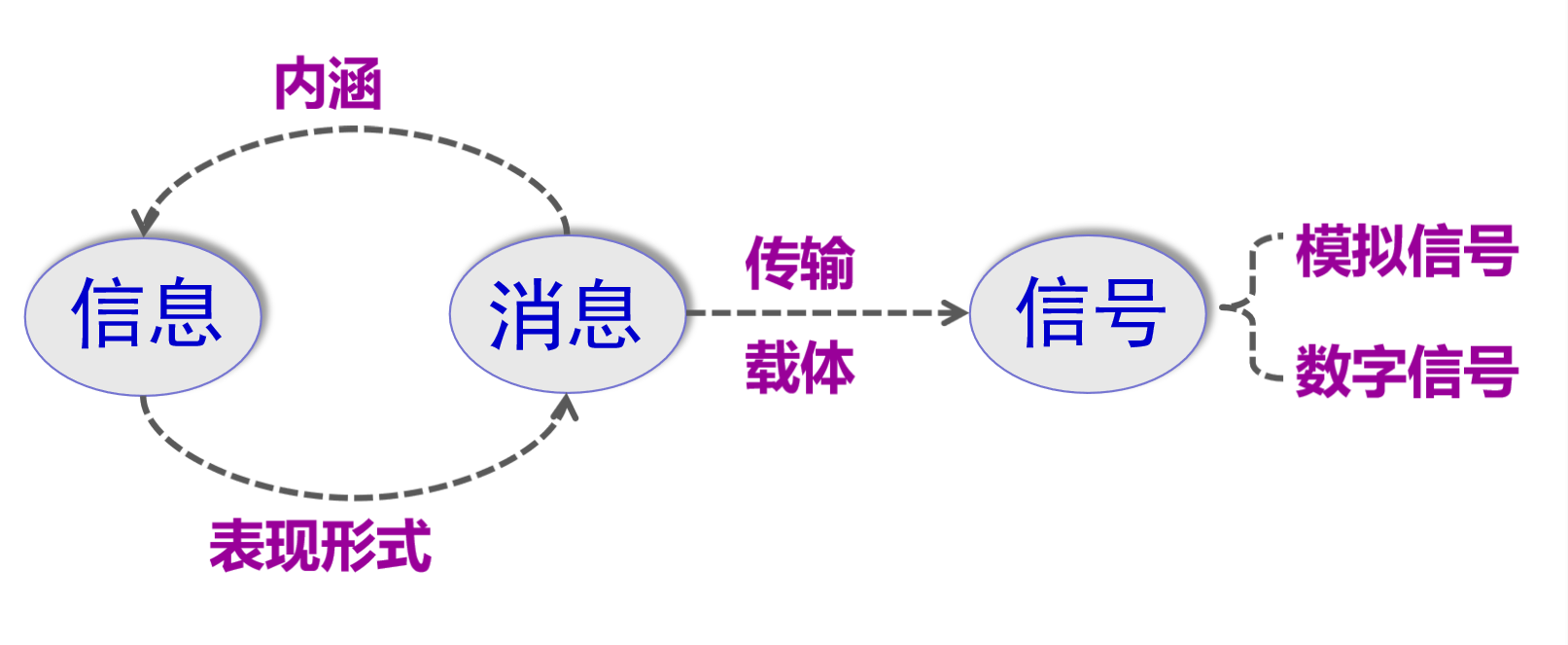即：消息是信息的物理形式、信息是消息的有效内容、信号是消息的传输载体
5）如何区分模拟信号和数字信号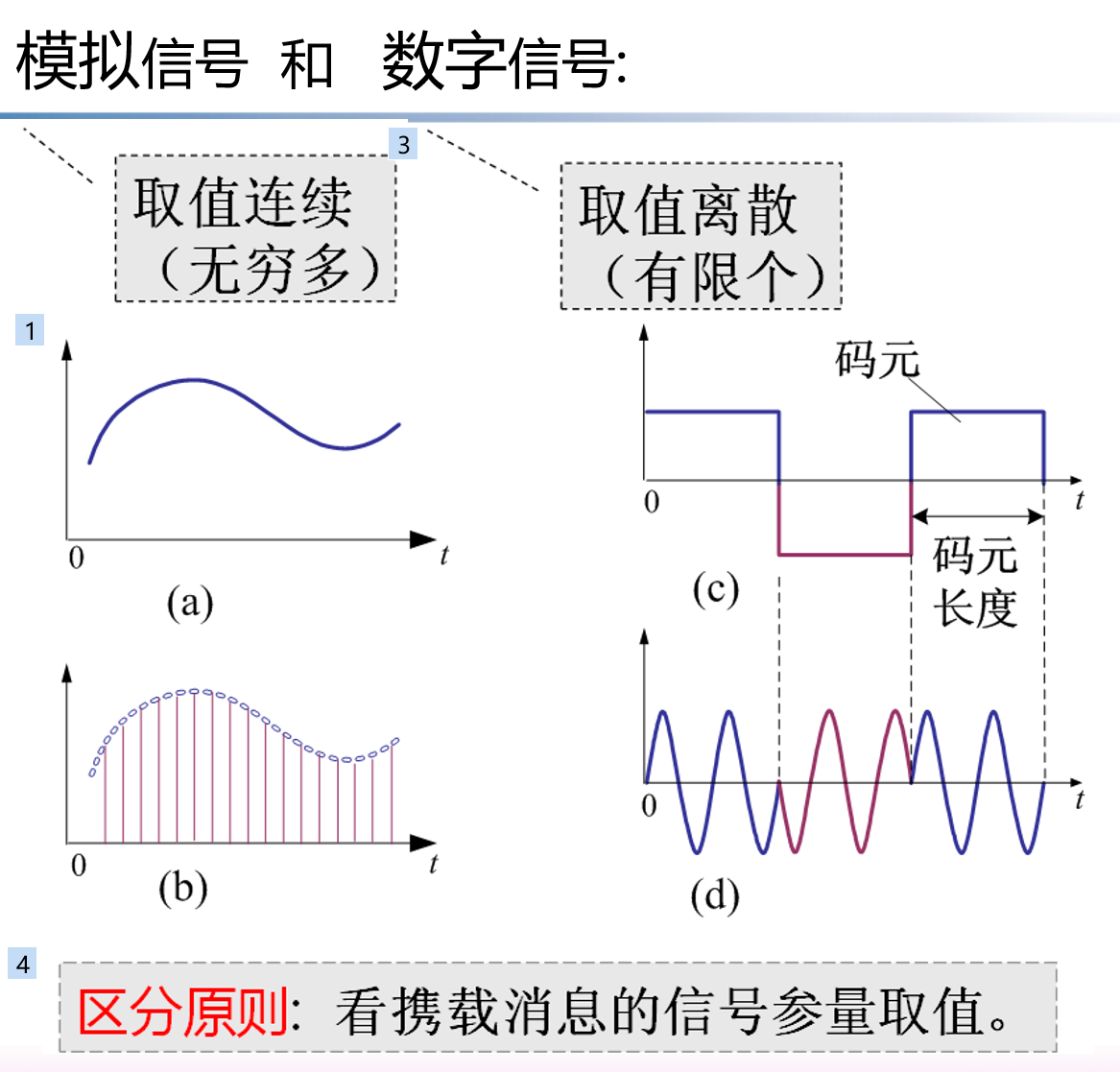6）如何将消息转换成电信号
通过信源（在下面通信系统模型会介绍到）来转换
如：话筒（声音传感器）把声音转变成音频信号；
数字终端把符号转变成数字信号；
摄像机把图像转变成视频信号；
热敏电阻（温度传感器）把温度转变成电信号。
7）通信：就是利用电信号传输消息中所包含的信息8
8）通信系统：完成通信过程所需要的电子设备和信道的总体
# 二、通信系统模型
1）通信系统的一般模型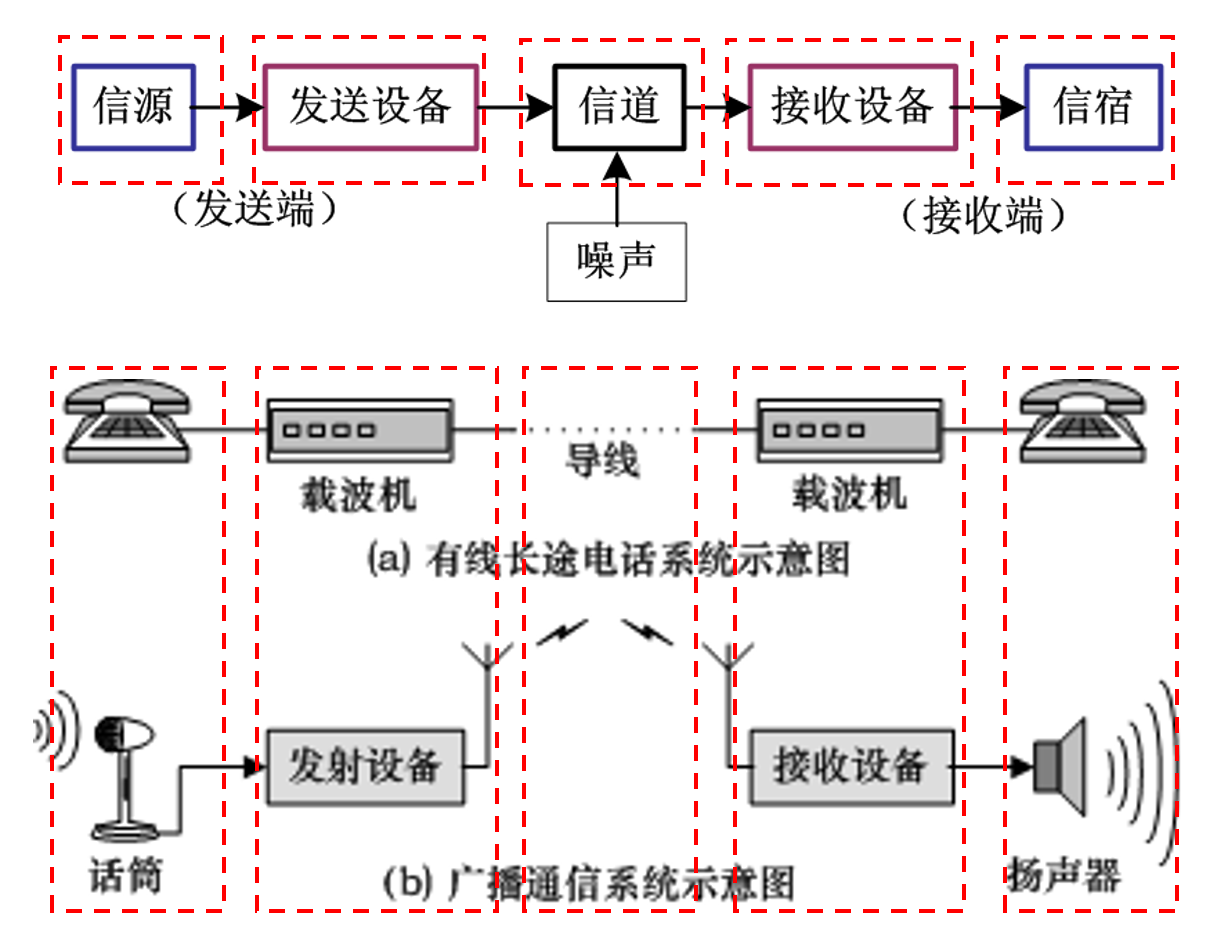1.信源：把消息转换成原始电信号。根据消息的种类不同，信源可分为模拟信源和数字信源。模拟信源输出连续的模拟信号，如话筒（声音->音频信号）；数字信源则输出离散的数字信号，如计算机等数字终端。
2.发送设备：作用是将原始电信号转变成适合在信道中传输的信号，使发送信号的特性和信道特性相匹配，具有抗信道干扰的能力，并且具有足够的功率以满足远距离传输的需要。发送设备包含变换、放大、滤波、编码、调制等过程。
3.信道：能传输信号的各种物理媒介，用来将来自发送设备的信号传送到接收端。在无线信道中，信道可以是自由空间；在有限信道中可以是明线、电缆和光纤。信道既给信号以通路，也会对信号产生各种干扰和噪声。
4.接收设备：功能是信号放大和反变换（如译码、解调等），目的是从受到减损的接收信号中正确恢复出原始电信号。
5.信宿：信宿是传送消息的目的地，功能是把原始电信号还原成相应的消息
2）模拟通信系统模型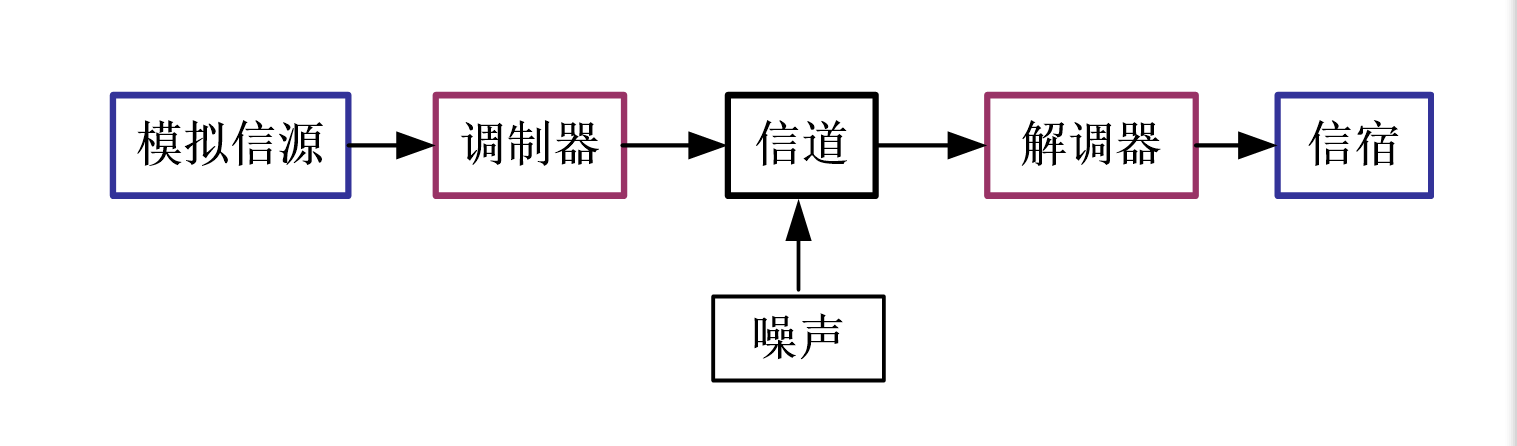两对重要的变换：
1.基带：在发送端把连续消息变换成原始电信号，在接收端进行相反的变换。这种变换和反变换是由信源和信宿来完成。以自由空间作为信道的无线电传输无法直接传输这些信号。
2.带通：把基带信号变成适合在信道中传输的信号，并在接收端进行反变换。
3）数字通信系统模型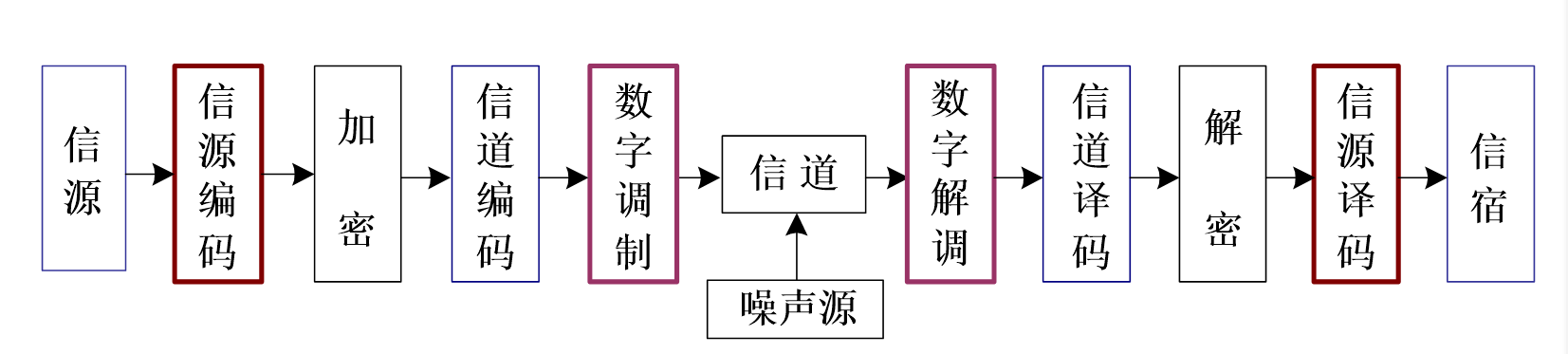1.信源编码与译码：
完成模数转换。即当信息源给出的是模拟信号时，信源编码器将其转换成数字信号，以实现模拟信号的数字传输。
提高信息传输的有效性。通过某种压缩编码技术设法减少码元数目以降低码元速率。
2.加密与解密：
人为的将被传输的数字序列扰乱，即加上密码。
在接收端利用与发送端处理过程相反的过程对收到的数字序列进行解密。
3.信道编码与译码：
差错控制，增强抗干扰能力，信道编码器对传输的信息码元按一定的规则加入保护成分。接收端的信道译码器按相应的逆规则进行解码，从中发现或纠正错误，提高通信系统的可靠性。
4.数字调制与解调：数字调制是把数字基带信号的频谱搬移到高频处，形成适合在信道中传输的带通信号。基本的数字调制方式有振幅键控、频移键控、绝对相移键控、差分相对键控。在接收端可以采用相干解调和非相干解调还原数字基带信号。
基本的
5.同步：使收发两端的信号在时间上保持步调一致。
4）数字通信特点
优点：1.抗干扰能力强且噪声不积累
2.传输差错可控
3.便于处理、变换、存储
4.便于将来自不同信源的信号综合传输
5.易于集成；易于加密
缺点：1.可能需要较大的传输带宽
2.对同步要求高
# 三、通信系统分类与通信方式
（1）通信系统分类方式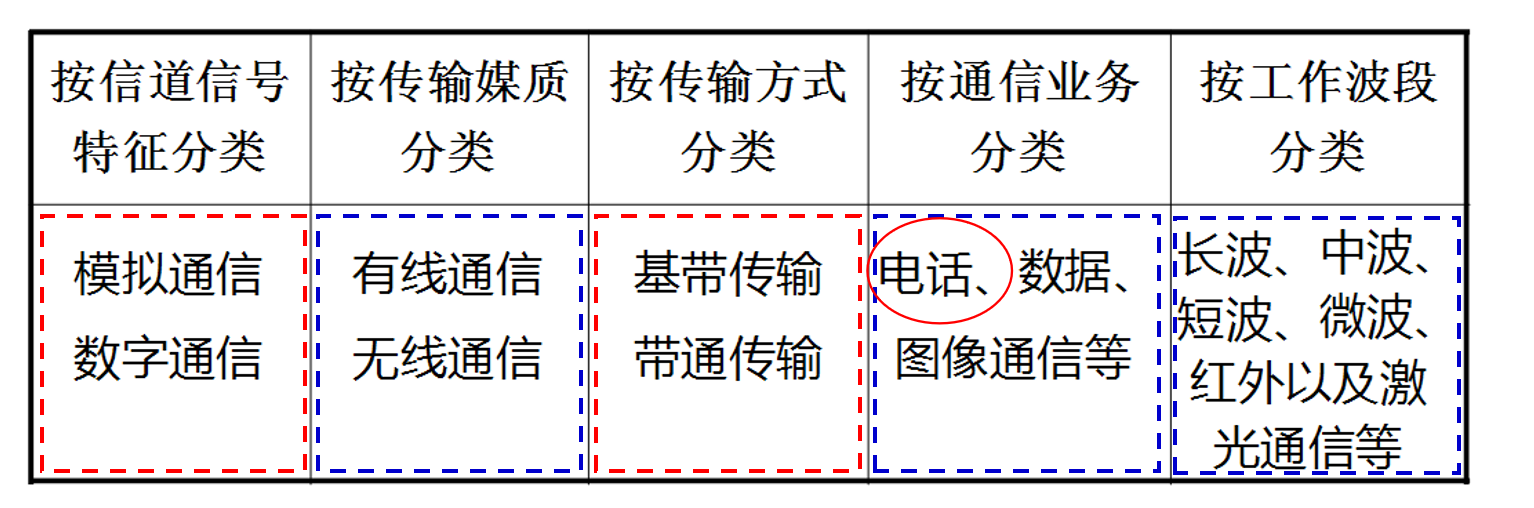按复用方式划分：频分、时分、码分复用
同一个通信方式可以分属不同分类。
（2）通信方式
1）按传输方向和时间分：单工、半双工和全双工通信2）按数字码元传输时序分：并行传输和串行传输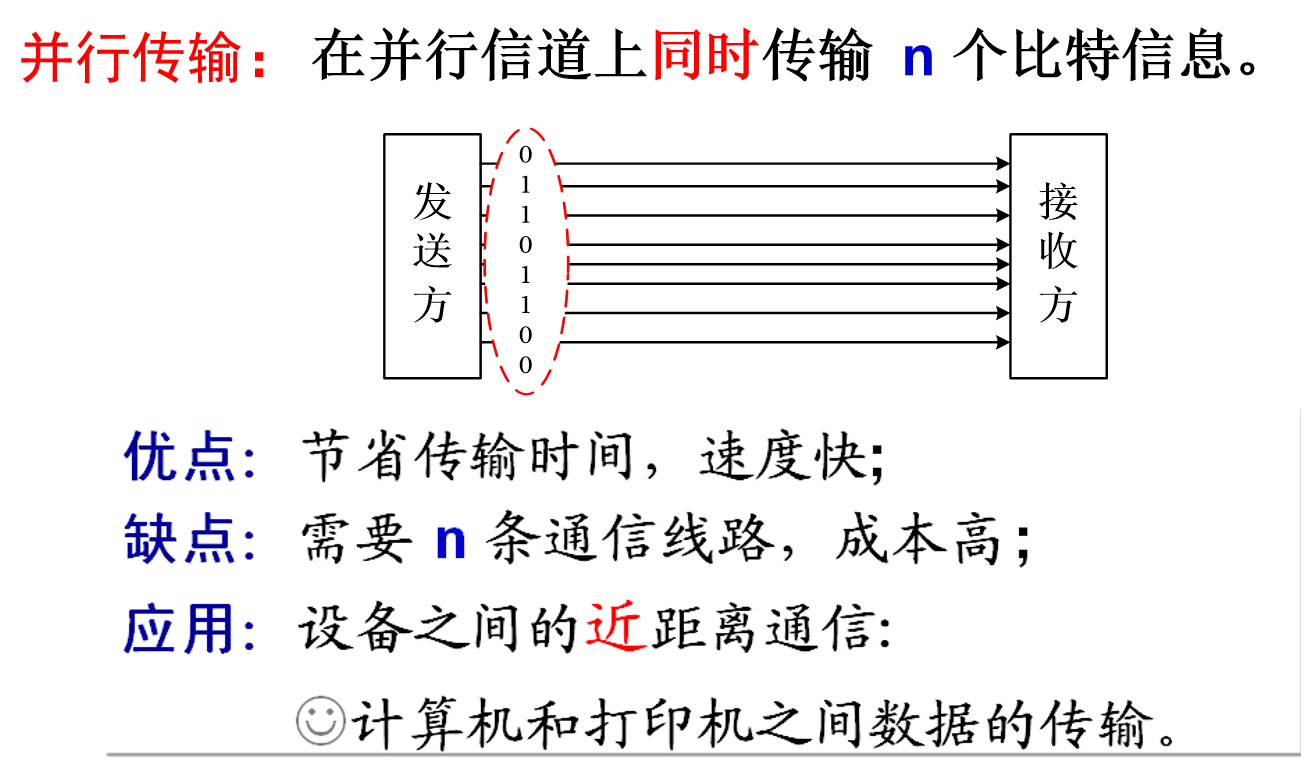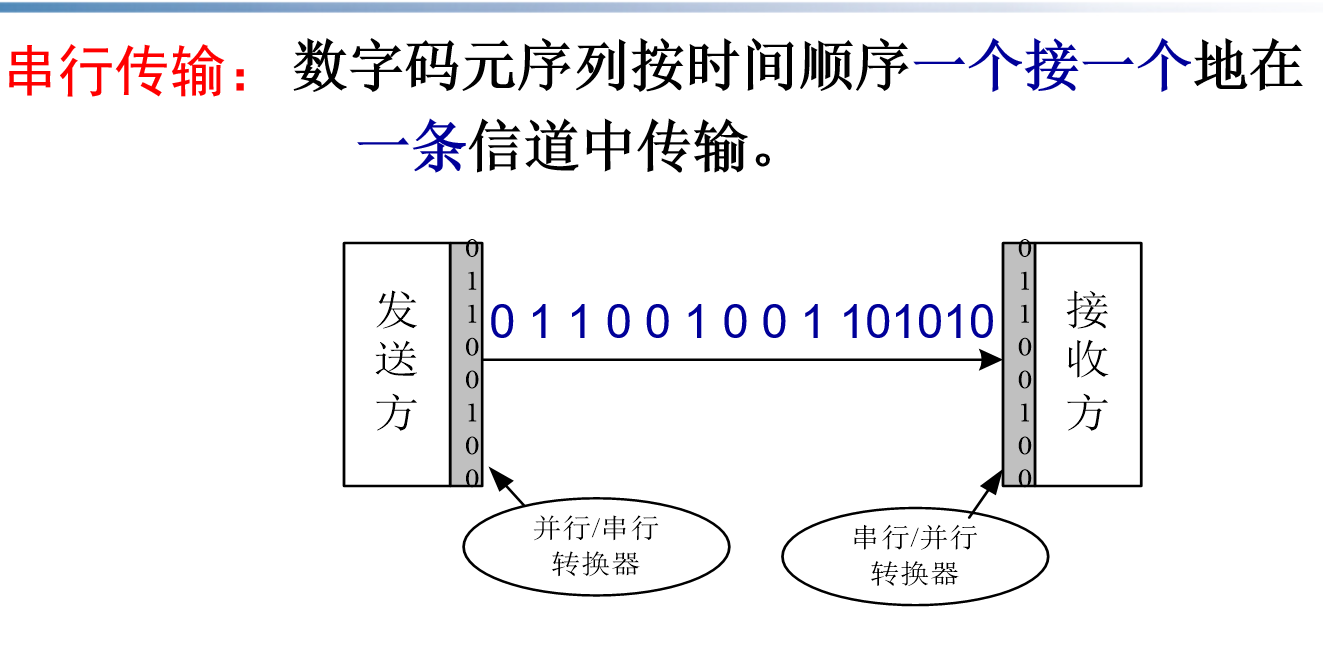展开全文• ## 移动通信系统

千次阅读 2020-03-01 13:19:45
第一次作业 1、什么是移动通信?移动通信有哪些主要特点?...2、移动通信系统有哪几部分组成?简述其主要作用。 移动交换子系统，操作维护管理子系统，基站子系统 1、完成信息交换、用户信息管理、呼叫接续、...
参考链接：
https://wenku.baidu.com/view/b3c472650b1c59eef8c7b4f5.html
http://www.doc88.com/p-495983025299.html
第一次作业
1、什么是移动通信?移动通信有哪些主要特点?
移动通信是指通信双方中至少有一方是处于运动 （或暂时停止运动）状态下进行的通信。
特点：（1）无线电波传播复杂（2）移动台受到的干扰严重 （3）无线电频谱资源有限（4）对移动设备的要求高（5）系统复杂
2、移动通信系统有哪几部分组成?简述其主要作用。
移动交换子系统，操作维护管理子系统，基站子系统
1、完成信息交换、用户信息管理、呼叫接续、号码管理等功能。
2、BSS系统是在一定的无线覆盖区中由MSC控制，与MS进行通信的系统设备，完成信道的分配、用户的接入和寻呼、信息的传送等功能。
3、MS是GSM系统的移动用户设备，它由两部分组成，移动终端和客户识别卡（SIM卡）。移动终端就是“机”，它可完成话音编码、信道编码、信息加密、信息的调制和解调、信息发射和接收。SIM卡就是“人”，它类似于我们现在所用的IC卡，因此也称作智能卡，存有认证客户身份所需的所有信息，并能执行一些与安全保密有关的重要信息，以防止非法客户进入网路。SIM卡还存储与网路和客户有关的管理数据，只有插入SIM卡后移动终端才能接入进网。
3、常用的移动通信系统包括哪几种类型?
（１）蜂窝式公用陆地移动通信系统 （蜂窝系统）
（２）集群移动通信系统
（3）无绳电话系统
（４）无线寻呼系统
（５）卫星移动通信系统
（６）无线 ＬＡＮ
4、FDD和TDD的概念及其各自的优势是什么?
频分双工 （FDD)：速度快，充分利用频谱。
时分双工（TDD）：能够灵活配置频率，使用FDD系统不易使用的零散频段;可以通过调整上下行时隙转换点，提高下行时隙比例，很好地支持非对称业务;具有上下行信道一致性，基站的接收和发送可以共用部分射频单元，降低了设备成本;接收上下行数据时，不需要收发隔离器，只需一个开关即可，降低了设备的复杂度;具有上下行信道互惠性，能够更好地采用传输预处理技术，如预RAKE技术、联合传输技术、智能天线技术等，能有效地降低移动终端的处理复杂性。
第二次作业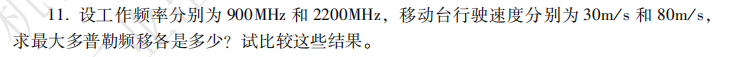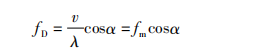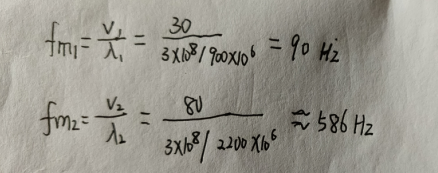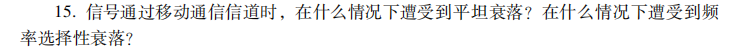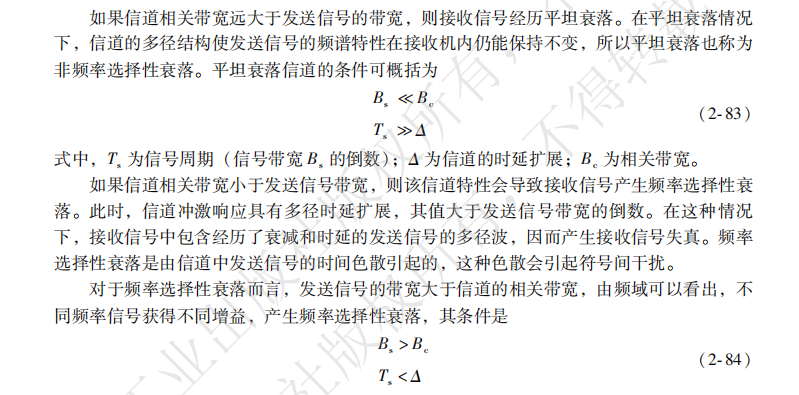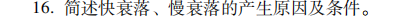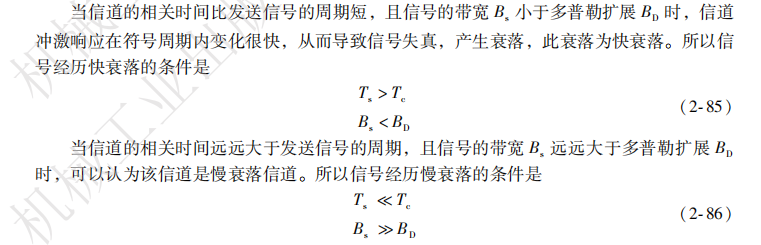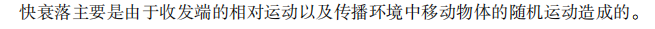第三次作业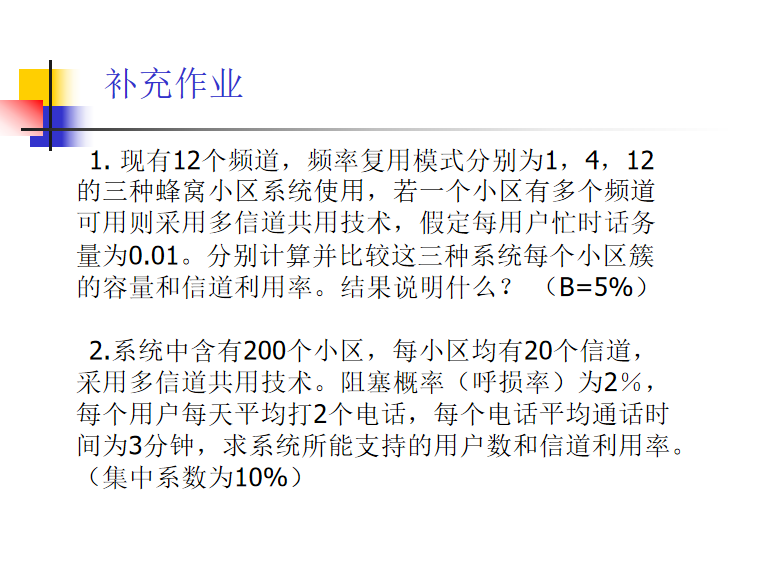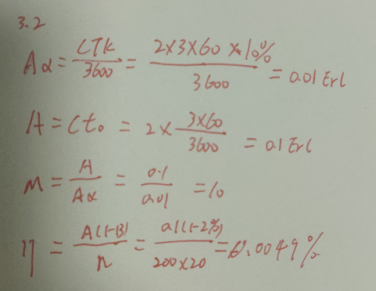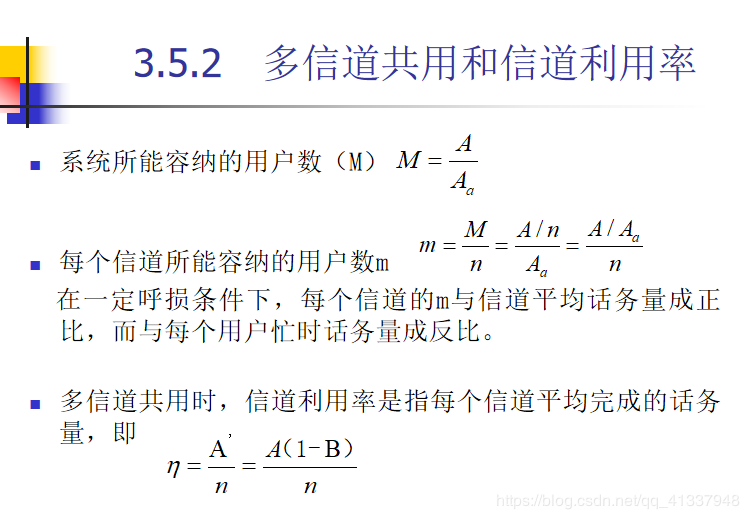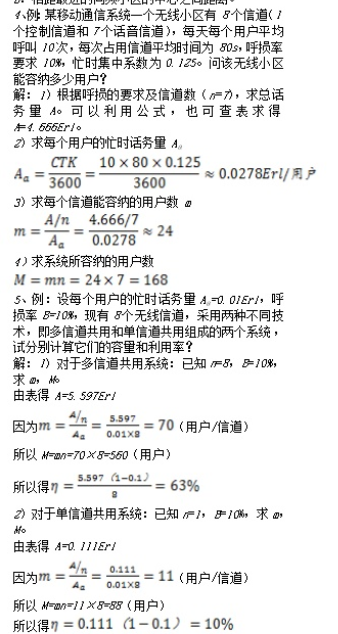展开全文• ## 通信系统概述

千次阅读 2019-07-23 20:46:56
一、通信系统组成：信息源、发送设备、信道、噪声源、接收设备、受信者。 信息源：将各种消息转换成原始电信号。信源可分为模拟信源和数字信源两种。 发送设备：产生适合于在信道中传输的信号。 信道：将来自...
一、通信系统组成：信息源、发送设备、信道、噪声源、接收设备、受信者。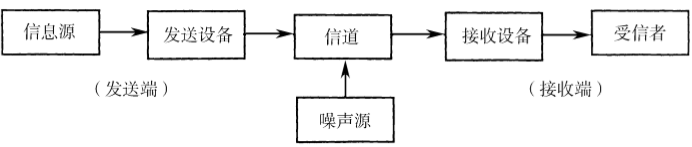信息源：将各种消息转换成原始电信号。信源可分为模拟信源和数字信源两种。

发送设备：产生适合于在信道中传输的信号。

信道：将来自发送设备的信号传送到接收端的物理媒介，分为有线信道和无线信道两大类。

噪声源：集中表示分布于通信系统中各处的噪声。

接收设备：从受到减损的接收信号中正确恢复出原始电信号。

受信者：把原始电信号还原成相应的消息。

二、通信系统的分类：

按照信道中传输的是模拟信号还是数字信号可以分为：模拟通信系统、数字通信系统。

按照传输媒介的不同可以分为：有线通信系统、无线通信系统。

按照工作波段的不同可以分为：长波通信、中波通信、短波通信。

按照信号复用方式可以分为：频分复用、时分复用、码分复用、空分复用。

按照信号调制方式可以分为：基带传输系统、带通(调制)传输系统。

按照信号通信方式可以分为：单工、半双工、全双工。

按照信号传输方式可以分为：并行传输、串行传输。

按照信号是否一致可以分为：同步通信系统、异步通信系统。

三、数字通信系统：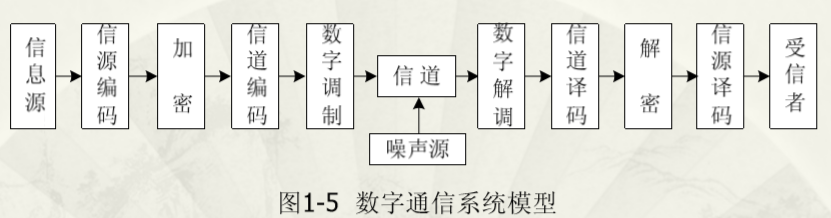信源编码与译码的目的：去除冗余信息，提高信息传输的有效性；完成模拟/数字的转换。

信道编码与译码的目的：进行差错控制，增强抗干扰的能力。

加密与解密的目的：保证传输信息的安全。

数字调制与解调的目的：形成适合在信道中传输的带通信号。

四、通信系统主要性能指标：有效性、可靠性。

有效性：指传输一定信息量时所占用的信道资源(频带宽度和时间间隔)，也就是传输的“速度”问题。

可靠性：指接收信息的准确程度，也就是传输的“质量”问题。模拟通信系统的可靠性用接收端最终输出信噪比(SNR)来度量。

频带利用率：是指单位频带(每Hz)内所实现的传输速率，可以表示为： 或 。频带利用率常常用于比较不同系统的传输效率，是评价数字通信系统有效性的重要指标之一。


展开全文信源编码 信道编码 性能指标
• 的水下自动生产系统，以及与国家安全有关的多种监控与观通等，它们要求现代的通信系统是：组成陆一海一空三维空间移动互联通信网。三维移动互联通信网的组成，当前主要制约于水下通信。通常我们往往都是通过连接电缆...
的水下自动生产系统，以及与国家安全有关的多种监控与观通等，它们要求现代的通信系统是：组成陆一海一空三维空间移动互联通信网。三维移动互联通信网的组成，当前主要制约于水下通信。通常我们往往都是通过连接电缆来实现水下通信。但有缆通信在应用中存在着很多弊端。比如，在深海、极地海域或恶劣海况下就很难开展工作，而电缆的“纠缠”问题更限制了潜器的机动灵活性，这些决定了水下通信要想摆脱这种类似“脐带”的束缚，必须向无缆的方向发展。

在陆地上，我们通常都是利用无线电方式进行无缆通信，但在水下，无

线电波的吸收和衰减都很大，只能以极低的发射频率(30 Hz．300 Hz)、大型天线和很高的发射功率进行工作，传输距离很短，无法完成水下通信任务。最新研究发展的蓝绿激光技术已取得了相当的进展，但因其造价昂贵，且作用距离小，不适用于大规模、长距离地水下通信。相比较看来，在我们所熟知的各种能量形式中，只有声波在水下的传播性能为最好，这使得以声波为载体的水声通信成为水下通信的主流。但是水声信道有如下特点：

1．有限通信带宽

由于声波在传播过程中的几何扩展及介质的粘滞、散射、反射、热传导

等物理吸收，引起声波能量的损失。这种传播过程中的能量损失随着通信系

统作用距离和工作频率的增加而增加，其中声吸收损失系数与声波频率的平

方成正比这些限制了水声通信系统的最大作用距离和最高频率，进而限制了系统的通信带宽。

2．多途效应

水声信道是缓慢时变的相干多途信道，在相干时间长度内，可简化为相干多途信道，仅存在多途效应。多途的形成与海洋环境和码元频率有关，其产生的机理有两种情况：在浅海信道，由于界面(海底、海面及目标)反射造成；而在深海，则主要由不同发射角的声线在传播过程中产生的弯曲造成，在某个区域，多条声线的相位达到一致，可形成会聚区。多途传播引起信号时问扩展。在浅海，多途扩展时间可达几百毫秒，而在深海扩展时间可至几秒。在所传送的码元间隔小于多途时间扩展的情况下，水声信道的多途效应将导致水声通信系统接收端信号中出现码间干扰ISI(Intersymbol Interference)，从而引起误码，降低系统的可靠性。多途效应是水声通信系统设计中最难克服的障碍，也是限制水声通信系统性能的主要因素。

3．海洋环境噪声和本地噪声

海洋环境噪声和本地噪声是水声通信的主要干扰背景，影响系统的接收信噪比，从而影响通信距离和可靠性。噪声和多途效应在远程和近程通信中对可靠性的影响不同：远程通信中，噪声和多途效应共同影响通信可靠性，而在近程通信中，多途效应的影响是主要的。

4．空变、时变、随机性

信道可被看为空变、时变的梳状滤波器，由若干子通带构成，每个子通带宽度只有几十～几百赫，其形状随时间和空间而变化。只有接收端实时感知信道的变化，才能有效的译码，所以，译码过程必需与信道的变化相匹配。信道编码也需考虑信道的空变、时变性。

5．多普勒效应

信道的时变、空变性，以及接收机与发射机之间的相对运动均可导致多普勒频移效应，它与收发点相对运动的速度及工作的频率成正比例关系。由于信号的多普勒频移，导致解码时系统检测能力降低，在某些情况下，为考虑多普勒容限，甚至还要降低系统的通信速率。概括地说，水声信道是一个带宽严重受限的缓慢时变、空变相干多途信道，传输条件十分恶劣，这些给水声通信的研究带来很大的难度。

Matlab简介：

Matlab是一种交互式的、以矩阵为基础的软件开发环境,它用于科学和工程的计算与可视化。Matlab的编程功能简单,并且很容易扩展和创造新的命令与函数。应用Matlab可方便地解决复杂数值计算问题。Matlab具有强大的Simulink动态仿真环境,可以实现可视化建模和多工作环境间文件互用和数据交换。Simulink支持连续、离散及两者混合的线性和非线性系统,也支持多种采样速率的多速率系统;Simulink为用户提供了用方框图进行建模的图形接口,它与传统的仿真软件包用差分方程和微分方程建模相比,更直观、方便和灵活。用户可以在Matlab和Simulink两种环境下对自己的模型进行仿真、分析和修改。用于实现通信仿真的通信工具包(Communication toolbox,也叫Commlib,通信工具箱)是Matlab语言中的一个科学性工具包,提供通信领域中计算、研究模拟发展、系统设计和分析的功能,可以在Matlab环境下独立使用,也可以配合Simulink使用。另外，Matlab的图形界面功能GUI（Graphical User Interface）能为仿真系统生成一个人机交互界面，便于仿真系统的操作。因此，Matlab在通信系统仿真中得到了广泛应用，本文也选用该工具对数字通信系统进行仿真。

信号可用来传输信息，是消息或数据的电磁编码。信息可用语言、文字、图象等表达，但在很多情况下，这些表达信息的语言文字不便于直接传输。因此在近代科学技术中，常用电信号来传送各种信息，即利用一种变换设备把各种信息转换为随时间作相应变化的电流或电压进行传输。这种随信息作相应变化的电压或电流就是电信号

通信系统可按照信道中传输的是模拟信号还是数字信号而分为模拟通信系统和数字通信系统。数字通信以其抗干扰能力强、无噪声累积、便于计算处理、便于加密、易于小型化、集成化等优势，成为当代通信领域的主流技术。

数字通信较之模拟通信有以下优点：

抗干扰能力强，无噪声积累
电信号在信道中传送时，会受到各种各样的电气干扰。在模拟通信中，这种干扰是很难消除的，使得通信质量变坏。而数字通信在接收端是根据收到的“ 1 ”和“ 0 ”这两个数码来判别的，一般不会影响通信质量。

保密性强，易于实现检错纠错
模拟信号在传送过程中能量会逐渐发生衰减使信号变弱，为了延长通信距离，要在线路上设立一些增音放大器。但增音放大器会把有用的信号和无用的杂音一起放大，杂音经过一道道放大以后，就会越来越大，甚至会淹没正常的信号，限制了通信距离。数字通信可采取“整形再生”的办法，把受到干扰的电脉冲再生成原来没有受到干扰的那样，使失真和噪音不易积累。

便于建立综合通信网
模拟通信传送的电信号，加密比较困难。而数字通信传送的是离散的电信号，很难听清。为了密上加密，还可以方便地进行加密处理。加密的方法是，采用随机性强的密码打乱数字信号的组合，敌人即使窃收到加密后的数字信息，在短时间内也难以破译。

设备可集成，微型化
数字通信的电路主要由电子开关组成，很容易采用各种集成电路，体积小、耗电少。

占用频带宽
数字通信的缺点是数字信号占用的频带比模拟通信要宽。一路模拟电话占用的频带宽度通常只有 4 千赫，而一路高质量的数字电话所需的频带远大于 4 千赫。但随着光纤等传输媒质的采用，数字信号占用较宽频带的问题将日益淡化。

数字通信将向超高速、大容量、长距离方向发展，新的数字化智能终端将产生。数字通信系统首先将模拟的语音信号经过模数转换为数字信号，其中经过抽样-量化-编码的过程。再对信号进行ASK调制，经过功率放大器后进行传输，接收端再进行相应的解调和译码，最终恢复出原始信号。

图中，信息源（也称发终端）可以把需要传输的消息经过转换变成原始电信号。信息源输出的信号我们通常称为基带信号。所谓基带信号是指没有经过调制（进行频谱搬移和变换）的原始电信号，其特点是频率较低，信号频谱从零频附近开始，具有低通形式。根据原始电信号的特征，基带信号可分为数字基带信号和模拟基带信号（相应地，信源也分为数字信源和模拟信源。）其由信源决定。

发送设备的功能是将信息和信道连接起来，即将信息源转换产生原始电信号（基带信号）再一次变换，使之可以在信道中传输。变换方式是多种多样的，比如调制适用于在需要频谱搬移的场合；对传输数字信号来说，发送设备又常常包含信源编码和信道编码等。
信道是指传送信号的物理性通道，可以是有线的，也可以是无线的，甚至还可以包含某些设备。图中的噪声源，是信道中的所有噪声以及分散在通信系统中其它各处噪声的集合。
在接收端，当接收设备收到由信道中传输的信号进行解调、译码、解码等。它的任务其实是发送设备的一个反过程，从带有干扰的接收信号中恢复出相应的原始电信号来。
受信者（也称信宿或收终端）是将复原的原始电信号转换成相应的消息，同样他也是信息源工作的一个反过程。

图中给出的是通信系统的一般模型，按照信道中所传信号的形式不同，可进一步具体化为模拟通信系统和数字通信系统。

语音信号的数字化，最简单的方法是对其直接进行模／数转换；只要采样率足够高，量化每个样本的比特数足够多，则可以保证解码恢复的语音信号有很好的音质，不会丢失用用信息。然而对语音信号直接数字化所需的码率太高，例如，普通电话通信中采用8kHz采样，8比特进行量化，数据率则达到64kbits／s。这样大的数据率即使对很大容量的传输信道也是难以承受的。尤其是在水声信道中，由于水介质的吸收使得可利用的工作频率较低，信道带宽较窄，因此，要想在水中获得高质量的数字语音通信就必须对语音信息进行大幅度的压缩，降低传输所需的比特率，这对实时语音通信尤为重要。目前，在语音编码领域，低速率语音编码体制(码速率在4．8Kb／s以下)因其广泛的需求而得到国内外研究者的重视。从技术角度讲，现代中低速语音编码体制主要有码本激励线性预测编码(CELP)和多带激励编码(MBE)。本系统采用CELP编码。CELP是Code Excited Linear Prediction的缩写。CELP是近10年来最成功的语音编码算法。CELP语音编码算法用线性预测提取声道参数，用一个包含许多典型的激励矢量的码本作为激励参数，每次编码时都在这个码本中搜索一个最佳的激励矢量，这个激励矢量的编码值就是这个序列的码本中的序号。但是由于其非常复杂，所以在此对A律13折线的PCM编码进行了仿真。

2)信道编码方式

我们所掌握的信道编码方式有两种，线性分组码和分组码。

线性分组码是把k个比特的序列编成n个比特的码组，每个码组的（n-k）个校验位仅与本码组的k个信息位有关，而与其他码组无关。为了达到一定的纠错能力和编码效率，分组码的码组长度通常都比较大。编译时会产生延时，随着n的增加而现行增加。

卷积码把k个信息比特编成n个比特，但k和n通常很小，特别适宜于以串行形式传输信息，延时小。编码后的k个码元既与前段信息有关，还与其余各段信息有关。卷积码的纠错能力随N增大而增大，但差错率降低。在编码器复杂性相同的情况下，卷积码的性能优于线性分组码。故本设计中采用卷积码来进行信道编码。

3）调制方式

水声信道的复杂特性是水声通信系统区别于其它通信系统，如无线电通信系统的最主要原因，它决定了水声通信系统的设计和性能。因而设计一个高效可靠的水声通信系统必须要考虑水声信道的特性。由于水声传播中信号的相位起伏较大，以往的水声通信系统都是采用非相干的调制方式，通过降低传输速率以获得较小的ISI，从而降低误码率，使用最多的是频移键控(FsK)调制方式。然而要在带宽严重受限的水声信道获得高传输速率，从理论上看这种非相干方式，无论系统的其他部分做得多么完善，在误码率容许的范围内，传输速率和带宽永远是一对无法调和的矛盾，从而大大制约了水声通信的质量。在这种情况下，人们不得不寻求能更有效利用带宽的调制方式。近年来的最新研究向人们展示了一个可喜的成果：相位相干调制法不仅能满足系统对数据率的要求，至少可获得十倍以上的增益，而且随着数据率的提高，信道的相干性变好，自适应接收器对信道的跟踪误差下降，从而有效地改善了接收性能。下表列出了一些利用相位相于调制技术的水声通信系统的最新研究成果（来源于网络）。

信道均衡技术是近年来在水声通信领域兴起的又一研究热点，使用以对

抗水声信道多途传播效应的有效途径之一。均衡的目的是在接收端对于信道非理想特性造成的信号畸变进行补偿，抑制接收信号之间的符号间干扰，从而尽可能的恢复发射波形。均衡器技术可以分为两类：线性均衡和非线性均衡。非线性均衡器包括判决反馈均衡器(DFE，Decision Feedback Equalizer)、最大似然符号检测器和最大似然系列估值器(MLSE，Maximum Likehood Sequence Estimator)。

均衡器的结构有横向滤波器型和格型等等。均衡器的算法有最小均方误差算法(LMS，Least Mean Square Error)、递归最小二乘法(RLS，Recursive Least

Square)、快速递归最小二乘法、平方根递归最小二乘法和递度最小二乘法等

等。下图就是一个简单的均衡器模型：

相关Matlab程序如下：

%%%%%%%%%%%%%%%%%%%%%%%%%%%%%%%%%%

%主程序

%卷积码的性能,(7,5), R=1/2

clear all;close all;

R=1/2;

EbN0dB = 0:1:6;

%BPSK调制

EsN0dB = EbN0dB + 10*log10(R);

N0 = 10.^(-EsN0dB/10); %求AWGN信道的单边功率谱密度

sigma = sqrt(N0/2);

%卷积码的结构

trellis = poly2trellis(3,[7,5]);

error = zeros(1,length(N0));

for k=1:length(N0)

n = 0;

while n<100 %每个信噪比下，仿真100帧

%信源产生

d1 = (sign(randn(1,1000))+1)/2;

d = [d1 zeros(1,2)]; %添加尾比特使编码后状态归0

%卷码编码

s = convencoder(d,trellis);

%BPSK、经过AWGN信道

r = (2*s-1) + sigma(k)*randn(1,length(s));

%译码

dd = viterbi(r,trellis);

%误码计数

error(k) = error(k) + sum( abs( dd(1:length(d1)) - d1) )

n = n+1 %计数仿了多少帧

end

ber(k) = error(k)/(n*1000);

end

semilogy(EbN0dB,ber);

%%%%%%%%%%%%%%%%%%%

%%%%%%%%%%%%%%%%%%%

%程序2

function [out] = convencoder(din,trellis)

%卷积码编码器

%输入：din {0,1}序列码流

% trellis Matlab格式网格结构(可以用poly2trellis获得)

%初态为0

curState = 0;

for k=1:length(din)

d(k) = trellis.outputs( curState+1,din(k)+1 );

curState = trellis.nextStates( curState+1, din(k)+1 );

end

N = floor( log2(trellis.numOutputSymbols) );

out = dec2bin(d,N)-48;

out = reshape(out',1,N*length(din));

%%%%%%%%%%%%%%%%%%%%%%%%%%%%%%%

%%%%%%%%%%%%%%%%%%%%%%%%%%%%%%%

%程序3

function [out] = viterbi(r,trellis)

%软判决译码方式，2PSK,

N = log2(trellis.numOutputSymbols);

L = length(r)/N;

numStates = trellis.numStates;

numInputs = trellis.numInputSymbols;

mem = log2(numStates);

cur_metric= zeros(1,numStates)+Inf;

cur_metric(1)=0;

next_metric = -ones(1,numStates);

path = zeros(numStates,L);

sur_path = zeros(numStates,L);

for k=1:L %译码时刻

for st = 1:numStates %状态

for i=0:numInputs-1 %输入

dout = dec2bin( trellis.outputs(st,i+1),N )- 48;

dout = 2*dout-1;

dist = sum( (r((k-1)*N+1:k*N) - dout ).^2 ); %计算欧式距离

nextState = trellis.nextStates(st,i+1) +1;

x = cur_metric(st) + dist;

if next_metric(nextState)<0 %判断下一状态是否新写入？

next_metric(nextState) = x;

sur_path(nextState,1:k) = [path(st,1:k-1) i];

else

if x < next_metric(nextState) %比较，输入支路的度量

next_metric(nextState) = x;

sur_path(nextState,1:k) = [path(st,1:k-1) i];

end

end

end

end

cur_metric = next_metric;

next_metric = -ones(1,numStates);

path = sur_path;

end

out = path(1,:);

得到的误码率图像如下图所示：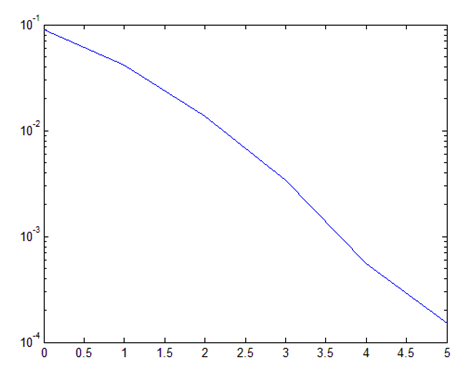仿真所得到的QPSK的频谱图如下：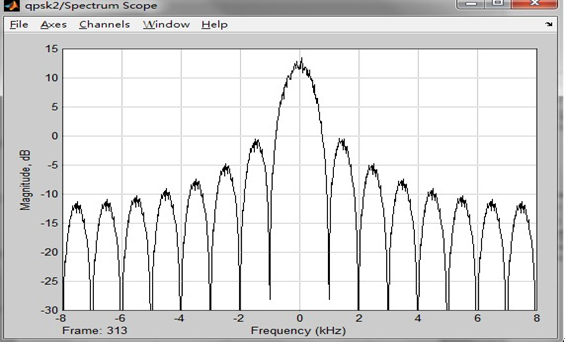QPSK调制后星座图如下：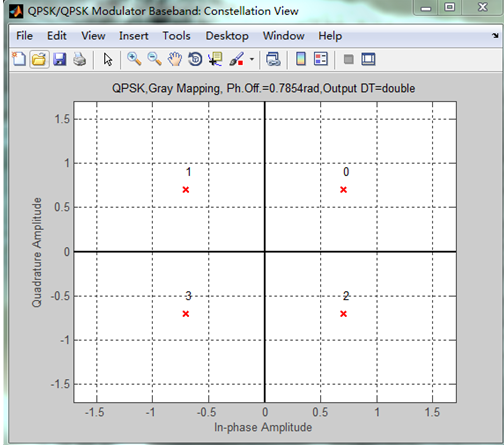QPSK信号通过高斯白噪声信道后得到的星座图如下：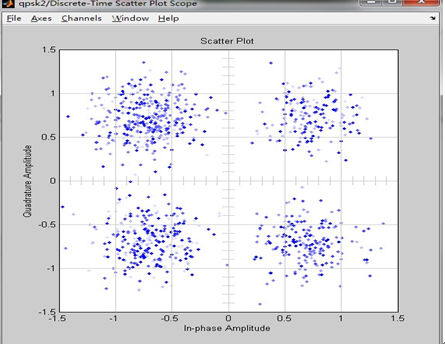用Matlab分析误码率和误比特率得到下图：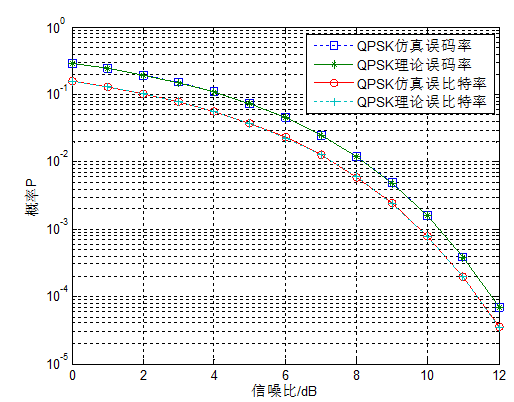此外，还用m文件对QPSK进行了理想信道，瑞利衰落信道，高斯白噪声信道下的仿真。

%主程序

clear;

%初始化参数

T=1;                          % 基带信号宽度，也就是频率

fc=10/T;                      % 载波频率

ml=2;                         % 调制信号类型的一个标志位（选取2的原因见23行）

nb=100;                       % 传输的比特数

delta_T=T/200;                % 采样间隔

fs=1/delta_T;                 % 采样频率

SNR=0;                        % 信噪比

t=0:delta_T:nb*T-delta_T;     % 限定t的取值范围

N=length(t);                  % 采样数

%  调制

% 基带信号的产生

data=randn(1,nb)>0.5;         % 调用一个随机函数（0 or 1），输出到一个1*100的矩阵

datanrz=data.*2-1;            % 变成极性码

data1=zeros(1,nb/delta_T);    % 创建一个1*nb/delta_T的零矩阵

for q=1:nb

data1((q-1)/delta_T+1:q/delta_T)=datanrz(q); % 将极性码变成对应的波形信号

end

% 将基带信号变换成对应波形信号

data0=zeros(1,nb/delta_T);    % 创建一个1*nb/delta_T的零矩阵

for q=1:nb

data0((q-1)/delta_T+1:q/delta_T)=data(q); % 将极性码变成对应的波形信号

end

% 发射的信号

data2=abs(fft(data1));

% 串并转换，将奇偶位数据分开

idata=datanrz(1:ml:(nb-1));   % 将奇偶位分开，因此间隔m1为2

qdata=datanrz(2:ml:nb);

% QPSK信号的调制

ich=zeros(1,nb/delta_T/2);    % 创建一个1*nb/delta_T/2的零矩阵，以便后面存放奇偶位数据

for i=1:nb/2

ich((i-1)/delta_T+1:i/delta_T)=idata(i);

end

for ii=1:N/2

a(ii)=sqrt(2/T)*cos(2*pi*fc*t(ii));

end

idata1=ich.*a;                % 奇数位数据与余弦函数相乘，得到一路的调制信号

qch=zeros(1,nb/2/delta_T);

for j1=1:nb/2

qch((j1-1)/delta_T+1:j1/delta_T)=qdata(j1);

end

for jj=1:N/2

b(jj)=sqrt(2/T)*sin(2*pi*fc*t(jj));

end

qdata1=qch.*b;               % 偶数位数据与余弦函数相乘，得到另一路的调制信号

s=idata1+qdata1;             % 将奇偶位数据合并，s即为QPSK调制信号

ss=abs(fft(s));              % 快速傅里叶变换得到频谱

% 瑞利衰落信道

ray_ich=raylrnd(0.8,1,nb/2/delta_T);

ray_qch=raylrnd(0.8,1,nb/2/delta_T);

Ray_idata=idata1.*ray_ich;

Ray_qdata=qdata1.*ray_qch;

Ray_s=Ray_idata+Ray_qdata;

% 高斯信道

s1=awgn(s,SNR);              % 通过高斯信道之后的信号

s11=abs(fft(s1));            % 快速傅里叶变换得到频谱

s111=s1-s;                   % 高斯噪声曲线

Awgn_s=awgn(Ray_s,SNR);         % 通过高斯信道再通过瑞利衰落信道

%QPSK 解调部分

% 解调部分（高斯信道）

idata2=s1.*a;                % 这里面其实隐藏了一个串并转换的过程

qdata2=s1.*b;                % 对应的信号与正余弦信号相乘

idata3=zeros(1,nb/2);        % 建立1*nb数组，以存放解调之后的信号

qdata3=zeros(1,nb/2);

% 抽样判决的过程，与0作比较，data>=0,则置1，否则置0

for n=1:nb/2

%     A1(n)=sum(idata2((n-1)/delta_T+1:n/delta_T));

if sum(idata2((n-1)/delta_T+1:n/delta_T))>=0

idata3(n)=1;

else idata3(n)=0;

end

%     A2(n)=sum(qdata2((n-1)/delta_T+1:n/delta_T));

if sum(qdata2((n-1)/delta_T+1:n/delta_T))>=0

qdata3(n)=1;

else qdata3(n)=0;

end

end

% 为了显示星座图,将信号进行处理

idata4=zeros(1,nb/2);

qdata4=zeros(1,nb/2);

for n=1:nb/2

Awgn_ichsum(n)=sum(idata2((n-1)/delta_T+1:n/delta_T))*delta_T;

if Awgn_ichsum(n)>=0

idata4(n)=1;

else idata4(n)=0;

end

Awgn_qchsum(n)=sum(qdata2((n-1)/delta_T+1:n/delta_T))*delta_T;

if Awgn_qchsum(n)>=0

qdata4(n)=1;

else qdata4(n)=0;

end

end

% 将判决之后的数据存放进数组

demodata=zeros(1,nb);

demodata(1:ml:(nb-1))=idata3; % 存放奇数位

demodata(2:ml:nb)=qdata3;     % 存放偶数位

%为了显示，将它变成波形信号（即传输一个1代表单位宽度的高电平）

demodata1=zeros(1,nb/delta_T);    % 创建一个1*nb/delta_T的零矩阵

for q=1:nb

demodata1((q-1)/delta_T+1:q/delta_T)=demodata(q); % 将极性码变成对应的波形信号

end

% 累计误码数

% abs(demodata-data)求接收端和发射端

% 数据差的绝对值，累计之后就是误码个数

Awgn_num_BER=sum(abs(demodata-data))

%%%%%%%%%%%%%%%%%%%

% 解调部分（瑞利+高斯）

Ray_idata2=Ray_s.*a;                % 这里面其实隐藏了一个串并转换的过程

Ray_qdata2=Ray_s.*b;                % 对应的信号与正余弦信号相乘

% Ray_idata3=zeros(1,nb/2);            % 建立1*nb数组，以存放解调之后的信号

% Ray_qdata3=zeros(1,nb/2);

% 抽样判决的过程，与0作比较，data>=0,则置1，否则置0

% for n=1:nb/2

%     if Ray_sum(Ray_idata2((n-1)/delta_T+1:n/delta_T))>=0

%        Ray_idata3(n)=1;

%     else Ray_idata3(n)=0;

%     end

%     if Ray_sum(Ray_qdata2((n-1)/delta_T+1:n/delta_T))>=0

%        Ray_qdata3(n)=1;

%     else Ray_qdata3(n)=0;

%     end

% end

% 为了显示星座图,将信号进行处理

Ray_idata4=zeros(1,nb/2);

Ray_qdata4=zeros(1,nb/2);

for n=1:nb/2

Ray_ichsum(n)=sum(idata2((n-1)/delta_T+1:n/delta_T))*delta_T;

if Ray_ichsum(n)>=0

Ray_idata4(n)=1;

else Ray_idata4(n)=0;

end

Ray_qchsum(n)=sum(qdata2((n-1)/delta_T+1:n/delta_T))*delta_T;

if Ray_qchsum(n)>=0

Ray_qdata4(n)=1;

else Ray_qdata4(n)=0;

end

end

% 将判决之后的数据存放进数组

Ray_demodata=zeros(1,nb);

Ray_demodata(1:ml:(nb-1))=Ray_idata4; % 存放奇数位

Ray_demodata(2:ml:nb)=Ray_qdata4;     % 存放偶数位

%为了显示，将它变成波形信号（即传输一个1代表单位宽度的高电平）

Ray_demodata1=zeros(1,nb/delta_T);    % 创建一个1*nb/delta_T的零矩阵

for q=1:nb

Ray_demodata1((q-1)/delta_T+1:q/delta_T)=Ray_demodata(q); % 将极性码变成对应的波形信号

end

% 累计误码数

% abs(demodata-data)求接收端和发射端

% 数据差的绝对值，累计之后就是误码个数

Ray_num_BER=sum(abs(Ray_demodata-data))

%

%%                误码率计算

%%      调用了cm_sm32();和cm_sm33(）函数

%%作用：  cm_sm32()用于瑞利信道误码率的计算

%%      cm_sm33()用于高斯信道误码率的计算

%% ecoh on/off 作用在于决定是否显示指令内容

%%%%%%%%%%%%%%%%%%%%%%%%%%%%%%%%%%%%%%%%%%%%%%%

SNRindB1=0:1:6;

SNRindB2=0:0.1:6;

% 瑞利衰落信道

for i=1:length(SNRindB1),

[pb,ps]=cm_sm32(SNRindB1(i));         % 比特误码率

smld_bit_ray_err_prb(i)=pb;

smld_symbol_ray_err_prb(i)=ps;

disp([ps,pb]);

echo off;

end;

% 高斯信道

echo on;

for i=1:length(SNRindB1),

[pb1,ps1]=cm_sm33(SNRindB1(i));

smld_bit_awgn_err_prb(i)=pb1;

smld_symbol_awgn_err_prb(i)=ps1;

disp([ps1,pb1]);

echo off;

end;

% 理论曲线

echo on;

for i=1:length(SNRindB2),

SNR=exp(SNRindB2(i)*log(10)/10);                 % 信噪比

theo_err_awgn_prb(i)=0.5*erfc(sqrt(SNR));        % 高斯噪声理论误码率

theo_err_ray_prb(i)=0.5*(1-1/sqrt(1+1/SNR));     % 瑞利衰落信道理论误码率

echo off;

end;

%%%%%%%%%%%%%%%%%%%%%%%%%%%%%%%%%%%%%%%%%%%%%

h = spectrum.welch;

% 第一部分（理想）

figure(1)

subplot(3,2,1);

plot(data0),title('基带信号');

axis([0 20000 -2 2]);

subplot(3,2,2);

psd(h,data1,'fs',fs),title('基带信号功率谱密度');

subplot(3,2,3);

plot(s),title('调制信号');

axis([0 500 -3 3]);

subplot(3,2,4);

psd(h,s,'fs',fs),title('调制信号功率谱密度');

subplot(3,2,5);

plot(demodata1),title('解调输出');

axis([0 20000 -2 2]);

subplot(3,2,6);

psd(h,demodata1,'fs',fs),title('解调输出功率谱密度');

%%%%%%%%%

% 通过高斯信道

figure(2)

subplot(2,2,1);

plot(s1),title('调制信号(Awgn)');

axis([0 500 -5 5]);

subplot(2,2,2);

psd(h,s1,'fs',fs),title('调制信号功率谱密度(Awgn)');

subplot(2,2,3);

plot(s111),title('高斯噪声曲线');

axis([0 2000 -5 5]);

subplot(2,2,4);

for i=1:nb/2

plot(idata(i),qdata(i),'r+'),title('QPSK信号星座图（Awgn）');hold on;

axis([-2 2 -2 2]);

plot(Awgn_ichsum(i),Awgn_qchsum(i),'*');hold on;

legend('理论值（发射端）','实际值（接收端）');

end

%通过高斯信道再通过瑞利衰落信道

figure(3)

subplot(2,2,1)

plot(Ray_s),title('调制信号(Ray+Awgn)');

axis([0 500 -5 5]);

subplot(2,2,2);

psd(h,Ray_s,'fs',fs),title('调制信号功率谱密度(Ray)');

subplot(2,2,3);

for i=1:nb/2

plot(idata(i),qdata(i),'r+'),title('QPSK信号星座图（Awgn+Ray）');hold on;

axis([-2 2 -2 2]);

plot(Ray_ichsum(i),Ray_qchsum(i),'*');hold on;

legend('理论值（发射端）','实际值（接收端）');

end

subplot(2,2,4)

semilogy(SNRindB2,theo_err_awgn_prb,'r'),title('误码率曲线');hold on;

semilogy(SNRindB1,smld_bit_awgn_err_prb,'r*');hold on;

semilogy(SNRindB2,theo_err_ray_prb);hold on;

semilogy(SNRindB1,smld_bit_ray_err_prb,'*');

xlabel('Eb/No');ylabel('BER');

legend('理论AWGN','仿真AWGN','理论Rayleigh','仿真Rayleigh');

%文件2

function [pb,ps]=cm_sm32(snr_in_dB)

% [pb,ps]=cm_sm32(snr_in_dB)

%                CM_SM3 finds the probability of bit error and symbol error for

%                the given value of snr_in_dB, signal to noise ratio in dB.

N=100;

E=1;                                            % energy per symbol

numofsymbolerror=0;

numofbiterror=0;

counter=0;

snr=10^(snr_in_dB/10);                          % signal to noise ratio

sgma=sqrt(E/snr)/2;                             % noise variance

s00=[1 0]; s01=[0 1]; s11=[-1 0]; s10=[0 -1];   % signal mapping

% generation of the data source

while(numofbiterror<100)

for i=1:N,

temp=rand;                                  % a uniform random variable between 0 and 1

if (temp<0.25),                             % with probability 1/4, source output is "00"

dsource1(i)=0; dsource2(i)=0;

elseif (temp<0.5),                          % with probability 1/4, source output is "01"

dsource1(i)=0; dsource2(i)=1;

elseif (temp<0.75),                         % with probability 1/4, source output is "10"

dsource1(i)=1; dsource2(i)=0;

else                                        % with probability 1/4, source output is "11"

dsource1(i)=1; dsource2(i)=1;

end;

end;

% detection and the probability of error calculation

for i=1:N,

ray=raylrnd(0.8);

n=sgma*randn(1,2);                          % 2 normal distributed r.v with 0, variance sgma

if ((dsource1(i)==0) & (dsource2(i)==0)),

r=ray*s00+n;

elseif ((dsource1(i)==0) & (dsource2(i)==1)),

r=ray*s01+n;

elseif ((dsource1(i)==1) & (dsource2(i)==0)),

r=s10*ray+n;

else

r=s11*ray+n;

end;

% The correlation metrics are computed below

c00=dot(r,s00); c01=dot(r,s01); c10=dot(r,s10); c11=dot(r,s11);

% The decision on the ith symbol is made next

c_max=max([c00,c01,c10,c11]);

if (c00==c_max), decis1=0; decis2=0;

elseif (c01==c_max), decis1=0; decis2=1;

elseif (c10==c_max), decis1=1; decis2=0;

else decis1=1; decis2=1;

end;

% Increment the error counter, if the decision is not correct

symbolerror=0;

if (decis1~=dsource1(i)), numofbiterror=numofbiterror+1; symbolerror=1;

end;

if (decis2~=dsource2(i)), numofbiterror=numofbiterror+1; symbolerror=1;

end;

if (symbolerror==1), numofsymbolerror=numofsymbolerror+1;

end;

end

counter=counter+1;

end

ps=numofsymbolerror/(N*counter);                          % since there are totally N symbols

pb=numofbiterror/(2*N*counter);                         % since 2N bits are transmitted

%文件3

function [pb1,ps1]=cm_sm32(snr_in_dB)

% [pb,ps]=cm_sm33(snr_in_dB)

%                CM_SM3 finds the probability of bit error and symbol error for

%                the given value of snr_in_dB, signal to noise ratio in dB.

N=100;

E=1;                                            % energy per symbol

snr=10^(snr_in_dB/10);                          % signal to noise ratio

sgma=sqrt(E/snr)/2;                             % noise variance

s00=[1 0]; s01=[0 1]; s11=[-1 0]; s10=[0 -1];   % signal mapping

% generation of the data source

numofsymbolerror=0;

numofbiterror=0;

counter=0;

while(numofbiterror<100)

for i=1:N,

temp=rand;                                  % a uniform random variable between 0 and 1

if (temp<0.25),                             % with probability 1/4, source output is "00"

dsource1(i)=0; dsource2(i)=0;

elseif (temp<0.5),                          % with probability 1/4, source output is "01"

dsource1(i)=0; dsource2(i)=1;

elseif (temp<0.75),                         % with probability 1/4, source output is "10"

dsource1(i)=1; dsource2(i)=0;

else                                        % with probability 1/4, source output is "11"

dsource1(i)=1; dsource2(i)=1;

end;

end;

% detection and the probability of error calculation

for i=1:N,

% the received signal at the detection, for the ith symbol,is:

n=sgma*randn(1,2);                          % 2 normal distributed r.v with 0, variance sgma

if ((dsource1(i)==0) & (dsource2(i)==0)),

r=s00+n;

elseif ((dsource1(i)==0) & (dsource2(i)==1)),

r=s01+n;

elseif ((dsource1(i)==1) & (dsource2(i)==0)),

r=s10+n;

else

r=s11+n;

end;

% The correlation metrics are computed below

c00=dot(r,s00); c01=dot(r,s01); c10=dot(r,s10); c11=dot(r,s11);

% The decision on the ith symbol is made next

c_max=max([c00,c01,c10,c11]);

if (c00==c_max), decis1=0; decis2=0;

elseif (c01==c_max), decis1=0; decis2=1;

elseif (c10==c_max), decis1=1; decis2=0;

else decis1=1; decis2=1;

end;

% Increment the error counter, if the decision is not correct

symbolerror=0;

if (decis1~=dsource1(i)), numofbiterror=numofbiterror+1; symbolerror=1;

end;

if (decis2~=dsource2(i)), numofbiterror=numofbiterror+1; symbolerror=1;

end;

if (symbolerror==1), numofsymbolerror=numofsymbolerror+1;

end;

end

counter=counter+1;

end

ps1=numofsymbolerror/(N*counter);                          % since there are totally N symbols

pb1=numofbiterror/(2*N*counter);                         % since 2N bits are transmitted

理想信道下的仿真，实验结果如图：

展开全文• ## 通信系统仿真

千次阅读 2016-06-19 20:21:45
实验目的这是一个综合性的大型实验，通过搭建一个包括信源、信源编译码器、信道、信道编译码器等各模块在内的仿真通信系统， 使学生能够加深对本课程各个重点章节的理解，更好地掌握通信的本质意义。说明： 由于...java
• ## 跳频通信系统仿真

千次阅读 2019-04-14 17:58:14
跳频通信系统仿真 源码： https://github.com/Grootzz/Frequency-Hopping 本示例演示了跳频通信系统的整个工作流程，包括调制，跳频，解跳，解调。 在本示例中，忽略同步相关的问题，包括跳频同步，帧同步，定时同步...matlab
• 一 通信的演进与热点（了解） ...同步：信息在通信系统中保持时间、空间、内容以及它们之间的同步。 2. 通信模型的基本元素 信源：信息产生的源头，可以是人或设备。其发出的信息也可以是多种多样
• 目录 文章目录目录4G 与 LTE...4G，即第四代移动通信系统，提供了 3G 不能满足的无线网络宽带化，主要提供数据（上网）业务。而 LTE（Long Term Evolution，长期演进技术）是电信领域用于手机及数据终端的高速无线通...
• 第 1章 MATLAB 基础与通信系统仿真 1.1 MATLAB 简介 1.1 1 MATLAB 的起源 1.1 2 MATLAB 的特点 1.2 MATLAB 程序设计 1.2.1 MATLAB 工作环境 1.2.2 MATLAB 的帮助系统 1.2.3 MATL店的基本操作 1.2.4 MATLAB 图形处理...matlab
• 相干光通信系统具有非常多的优点，广泛运用在长距离光传送网中。近年来，随着网络流量的爆炸式增长，数据中心对数据传输速度也提出了新的要求，相干光通信在数据中心短距光互连中也体现出来了许许多多的优势，得到...
• 光纤通信系统组成以及MATLAB实现 第一次写博客，主要想总结一下如何将光纤通信中的重要硬件组成和软件实现相结合。主要包括光纤通信系统的主要组成合如何用MATLAB对信号的产生和接收进行模拟。主要目的为自己总结给...MATLAB
• 共22讲，讲解如何实现一个仿腾讯QQ即时通信系统的课程；主要功能有：用户注册，登录，登录后QQ号码保存在登录下拉框中；修改个人资料，查找好友，添加好友，删除好友；在线聊天，上线通知，下线通知，更改状态，更换...编程语言 c语言编程软件 编程语言排行榜
• 无人机通信系统知识点总结 一、信号频段 5.8G：该频段暂时是航拍领域使用最广泛的无线微波视频传输器。 优点：体积轻盈，低热量，距离远，并且价位很透明，频道最大可释放出32个可选。至今仍是航拍微波传图领域的...
• 实验二 CDMA通信系统仿真 实验目的 1、掌握基于CDMA通信系统收发机的设计以及CDMA信号在AWGN和Rayleigh信道的误码率。 2、理解对通信系统性能产生影响的因素以及在不同信道环境下的系统性能。 实验仪器 ...
• ## 仿QQ即时通信系统

千次阅读 2018-07-12 22:45:04
这学期学了网络编程，课设是让做一个通信系统，能力有限，想不出来自己能设计一个什么通信系统，就模仿腾讯的产品设计一个仿QQ的通信系统。PS：其实背景可以改一下，XX公司需要一个内部通信系统… 话不多说，先上...
• 前面几节介绍了相干解调的几个基本概念，下来结合一个实际的通信系统，从实际的应用出发深入理解载波同步的概念。载波同步是基于锁相环技术使本地获取和载波同频同相的参考信号，用来解调信号。 载波同步就是对本地...QPSK解调
• 从模拟通信到数字通信，从计算机通信到移动通信，从有线通信到无线通信，从地面通信到卫星通信，从宏观电磁波通信到微观量子通信，从语音通信到视频通信，从...通过不同通信系统的案例，阐述其内在基本原理与主要技术。
• 卫星移动通信系统的各种分类方式 卫星移动通信系统的分类可按其应用来分， 也可以按他们所采用的技术手段来分。 （1） 按应用分类 可分为海事卫星移动系统（MMSS） 、 航空卫星移动系统（AMSS） 和陆地卫星移动系统...
• ## RFID通信系统框图

千次阅读 2015-04-23 21:44:12
RFID通信系统图http://img.blog.csdn.net/20150423214142140
• AUTOSAR架构的通信系统研究 文章介绍了AUTOSAR体系架构，对AUTOSAR COM规范基于信号的通信机制及其特有的传输模式切换系统进行了分析，旨在实现一种符合AUTOSAR COM规范的通信系统。 AUTOSAR体系架构 AUTOSAR即...
• ## vsat卫星通信系统

千次阅读 2014-09-26 09:33:47
【什么是VSAT卫星通信系统】 　VSAT是VERY SMALL APERTURE TERMINAL的缩字，直译为甚小口径卫星终端站。所以也称为卫星小数据站（小站）或个人地球站（PES），这里的“小字”指的是VSAT卫星通信系统中小站设备的...
• ## 数字通信系统模型

千次阅读 2015-04-18 10:45:21
数字通信系统模型如图1.2所示。图中信源把原始消息变换成原始电信号。常见的信源有产生模拟信号的电话机话筒、摄像机输出的视频模拟信号等。下面分别介绍数字通信系统模型中各个方框应完成的任务和所起的作用。 ...
• ## 通信系统之时分复用

千次阅读 多人点赞 2018-06-19 22:13:20
本博文上承接上篇博文，如下：通信系统之信源编码————————————————————————————————————————————————————一路基带话音信号的最高频率为3.4KHz，一般取 ：那么...
• 一、概述  当前，突发安全生产...本项目卫星通信系统建设主要包括卫星地面中心站通信系统、静中通应急指挥车卫星通信系统两大部分。  二、建设目标与原则 2.1 建设目标  1、建设XXX卫星地面中心站通信系统、...
• 直接扩频通信具有低截获概率、抗干扰能力强以及易于实现码分多址等优点，在抗干扰通信及民用移动通信中都得到了广泛的应用。 仿真流程图 用户1扩频加扰调制高斯信道walsh码扩频M序列加扰载波调制高斯白噪声高斯信道...Matlab
• 随着数字通信系统的发展，语音通信已成为人们日常生活的一部分，手机，电话和网络语音通信等已经逐渐深入人们的日常生活，通信技术是信息技术中极重要的组成部分。 语音是人体发音器官通过声带抖动发出的具有特殊...
• 现在需要做一个高并发多线程通信系统，打算用Java写IOCP服务端，实现数据的收发，以前只写过基于socket的 i/o通信模型，iocp 的没写过，希望打大家分享下用Java实现iocp的demo，谢谢iocp java 高并发
• 一般来说，无线通信系统物理层主要包括CRC、信道编码、加扰、调制、MIMO 、OFDM、扩频、组帧、上采样/上变频，接收端是发射端的逆过程，主要包括下采样、同步、OFDM解调、解扩、信道估计与均衡、MIMO检测、解调、解...物理层
• ## 通信系统的组成（精简介绍）

万次阅读 多人点赞 2018-06-17 20:01:30
古代的通信方式：（故事太动人，一个科普性的东西把我看的差点老泪纵横）通信系统的组成：信源与信宿的作用：信源将原始信号（声音、图像等）转化为电信号，经过发送设备发送，经过信道，由接受设备接受，到达信宿，...
• 随着通信技术的不断发展，现有的通信系统已经无法满足人们各个方面的需求，为了解决这个问题，5G通信技术应运而生。5G技术拥有更高的通信传输速率，更大的通信范围以及更高的频谱使用效率。5G通信技术的关键是毫米......